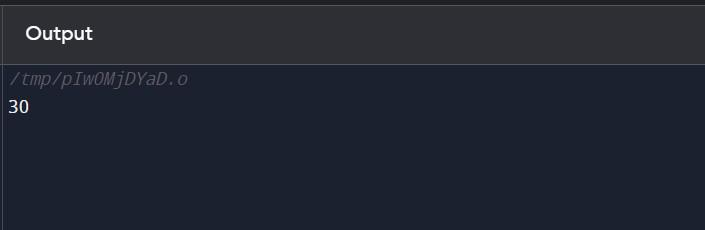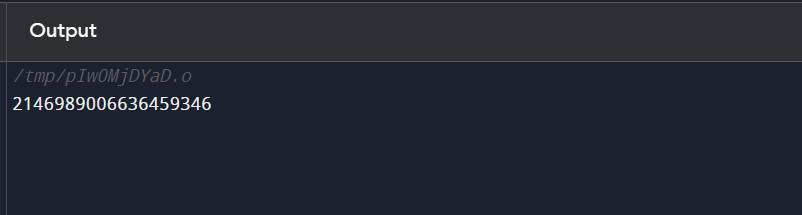Related Articles

# Solidity – Conversions

Solidity is a programming language that is used to write smart contracts for the Ethereum blockchain. One important concept in Solidity is conversions, which allow you to change the type of a variable or expression. The article focuses on discussing three types of conversions in Solidity.

The following conversions will be discussed here:

1. Implicit Conversions.
2. Explicit Conversions.
3. Conversions between Literals and Elementary Types.

Let’s start discussing each of these conversions in detail.

## Implicit Conversions

These occur automatically when a variable or expression of one type is assigned to a variable of a different type. For example, an integer can be implicitly converted to a fixed point number or a string. These are performed using built-in functions such as ‘uint()’, and ‘int()’.

Here is the syntax for some of the common conversion functions in Solidity:

### 1. uint()

Converts an expression to an unsigned integer (uint).

Example:

bytes memory b = “Hello World”;

uint a = uint(b); // a is now 7210465

### 2. int()

Converts an expression to a signed integer (int).

Example:

bytes memory b = “Hello World”;

int a = int(b); // a is now 7210465

### 3. bytes()

Converts an expression to a byte array (bytes).

Example:

uint a = 10;

bytes b = bytes(a); // b is now [0x0a]

Converts an expression to an address type.

Example:

bytes memory b = “0x742d35Cc6634C0532925a3b844Bc454e4438f44e”;

### 5. bool()

Converts an expression to a boolean type.

Example:

bytes memory b = “Hello World”;

bool a = bool(b); // a is now true

The below code demonstrates an implicit conversion from a ‘uint’ to an ‘int’:

## Solidity

 `// Solidity program to demonstrate ` `// an implicit conversion from a  ` `// 'uint' to an 'int' ` `pragma solidity ^0.8.0; ` ` `  `contract` `ImplicitConversion { ` `    ``function add() ``public` `pure returns (uint) { ` `        ``uint a = 10; ` `        ``uint b = 20; ` `        ``return` `a + b; ` `    ``} ` `} `

Explanation:

In this example, the function add() declares two variables a and b of type uint and assigns them the values 10 and 20 respectively. Then it returns the sum of both variables. The sum of these variables is implicitly converted to uint as both variables are of uint type.

Output:## Explicit Conversions

These are performed using built-in functions such as ‘bytes()’. These functions allow you to convert a variable or expression to a specific type. Explicit conversions are performed using type casts.

### 1. Integer Converted to Smaller Type

If an integer is converted to a smaller type then the higher-order bits are cut-off.

uint32 a = 0x432178;

uint16 b = uint16(a); // b will be 0x2178 now

### 2. Integer Converted to Larger Type

If an integer is explicitly converted to a larger type, it is padded on the left.

uint16 a = 0x4356;

uint32 b = uint32(a); // b will be 0x00004356 now

### 3. Fixed-size Bytes Converted to Smaller Types

Fixed-size bytes when converted to smaller types will cut off the sequence.

bytes2 a = 0x4326;

bytes1 b = bytes1(a); // b will be 0x43

### 4. Fixed-size Bytes Converted to Larger Types

Explicitly converting fixed-size bytes to a larger type, it is padded on the right.

bytes2 a = 0x4235;

bytes4 b = bytes4(a); // b will be 0x42350000

### 5. Explicit Conversion Between Integers and Fixed-size Byte Arrays

Explicit conversions between integers and fixed-size byte arrays are allowed only if both have the same size. Intermediate conversions are required to convert between integers and fixed-size byte arrays of different sizes.

bytes2 a = 0x3423;

uint32 b = uint16(a); // b will be 0x00003423

uint32 c = uint32(bytes4(a)); // c will be 0x34230000

uint8 d = uint8(uint16(a)); // d will be 0x23

uint8 e = uint8(bytes1(a)); // e will be 0x34

The below code demonstrates explicit conversion from a string literal to a bytes variable:

## Solidity

 `// Solidity program to demonstrate ` `// explicit conversion ` `pragma solidity ^0.8.0; ` ` `  `contract` `ExplicitConversion  ` `{ ` `function convert() ``public` `pure returns (bytes memory) { ` `        ``string memory str = ``"Hello World"``; ` `        ``bytes memory b = bytes(str); ` `        ``return` `b; ` `} ` `}`

Explanation:

In this example, the function convert() declares a variable b of type bytes memory and assigns it the value “Hello World”. Then it uses the bytes() function to explicitly convert the value of b to a byte type, and it returns the converted value. The output of the above code will be a single number, the result of converting the bytes of the string “Hello World” to an unsigned integer.

Output:## Conversions between Literals and Elementary Types

### 1. Integer Types

Decimal and hexadecimal literals can be implicitly converted to any integer type that is large enough to represent the literal without any truncation.

Valid:

unit8 a = 23;

uint32 b = 2134;

Invalid:

uint16 c = 0x123456;

Error: Literal is too large to fit in unit16.

### 2. Fixed-Size Byte Arrays

Decimal number literals cannot be implicitly converted to fixed-size byte arrays but hexadecimal number literals can be converted to fixed-size byte arrays but only if the number of hex digits exactly fits the size of the byte type. Decimal and hexadecimal number literals that have a value of zero can be converted to any fixed-size bytes type.

Valid:

bytes2 a = 0x1234;

bytes2 b = 0;

Invalid:

bytes2 a = 54321;

bytes2 b = 0x123;

String literals and hex string literals can be implicitly converted to fixed-size byte arrays only if the number of characters matches the size of the byte type.

Valid:

bytes2 a = hex”1234″;

bytes2 b = “xy”;

Invalid:

bytes2 a = hex”12″;

bytes2 b = “xyz”;